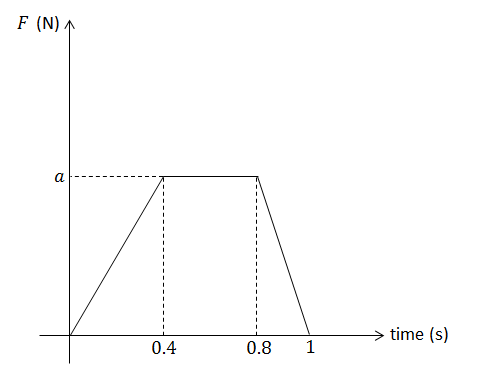Classical Mechanics

# Impulse of a variable force

A crate of mass $2\text{ kg}$ lies at rest on a floor. The coefficient of static friction between the crate and floor is $\mu_s=0.3,$ and that of kinetic friction between the crate and floor is $\mu_k=0.1.$ If a horizontal force $F$ is applied on the crate, and the magnitude of the force changes with respect to time $t$ according to the equation $F=3t$ in unit of N, what is the speed of the crate after four seconds?

Assume that gravitational acceleration is $g=10\text{ m/s}^2.$

A crate of mass $2.6\text{ kg}$ is initially at rest on a frictionless inclined plane that makes a $30^\circ$ angle with the horizontal. A force $F$ whose magnitude is $F=(18-t)\text{ N}$ with respect to time $t$ is exerted on the crate for five seconds in the direction up the plane. Find the approximate resulting velocity of the crate.

Assume that gravitational acceleration is $g=10\text{ m/s}^2.$

An object of mass $2\text{ kg}$ is moving on a frictionless horizontal floor with a velocity of $1.4\text{ m/s},$ in a straight line. If two consecutive forces $F_1=2\text{ N}$ and $F_2=-6\text{ N}$ are applied on the object for $7$ and $8$ seconds each, respectively, what is the resulting velocity of the object?

An airplane of mass $5000\text{ kg}$ is flying straight at a constant speed of $70\text{ m/s}.$ A head wind starts to blow, and the force exerted by the wind increases $50\text{ N}$ in magnitude every second. If the plane propels itself at a constant power, how much speed will the plane lose after ten seconds?An object of mass $5\text{ kg},$ initially at rest, is pushed by a force $F$ of duration $1\text{ s}$ whose strength varies with time according to the graph above. If $a=4,$ what is the resulting speed of the object?

×